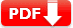# ASVAB Mechanical Comprehension Practice Test 2021 (Free PDF)

ASVAB Mechanical Comprehension Practice Test 2021 Download ASVAB Mechanical Quiz Test for Armed Services Vocational Aptitude Battery exam preparation online. Download printable and editable PDF files for ASVAB Mechanical Comprehension Practice Test.

Mechanical Comprehension (MC) tests comprehension of the principles Of mechanical devices and properties Of materials. Mechanical comprehension topics include simple machines, compound machines, mechanical motion, fluid dynamics, properties of materials, and structural support.

Actual Exam Content in MC Part

 Test Type Number of Question ASVAB Paper-and-Pencil 25-items CAT-ASVAB Test 16-items

## ASVAB Mechanical Comprehension Practice Test 2021

The following ASVAB Mechanical Comprehension (MC) Practice Test is only for reference purpose, it’s not an actual exam test questions. You can download our free ASVAB Mechanical Comprehension Practice Question in PDF (printable and editable), this will help you to make better ASVAB Test Prep offline. You can also use these files (PDF, DOC) as worksheets.

 Name of the Test ASVAB Practice Test ASVAB Stands for Armed Services Vocational Aptitude Battery AFQT Stands for Armed Forces Qualification Test Test Type Sample Multiple Choice Test Total Questions 20 (twenty) Answers and Explanation Available Printable and Editable PDF Available Subject Name Mechanical Comprehension (MC) Purpose Measures knowledge of the principles of mechanical devices, structural support, and properties of materials

## ASVAB Mechanical Practice Test 2020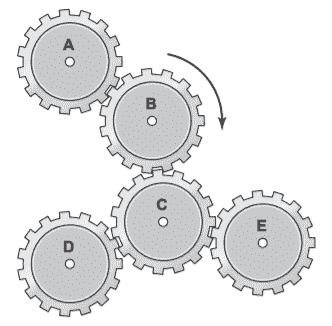1. The diagram shows five gears. If gear B turns as shown, then the gears turning in the same direction are

• A. A and C.
• B. A and D.
• C. C and D.
• D. D and E.

In this gear system, gear B is turning clockwise. That means that gears A and C are turning counter clockwise. Since gear C is turning counterclockwise, gears D and E are turning clockwise, the same direction as gear B.

2. What is the theoretical mechanical advantage in using this lever?• A. 1
• B. 2
• C. 3
• D. 4

For a class 1 lever, mechanical advantage is calculated as follows: MA = effort distance/load distance. In this case, MA = 18/6  3. /su_spoiler]

3. A wrench is used to turn a bolt that has 20 threads per inch. After 15 complete turns of the wrench, the bolt will have moved

• A. 1⁄4 inch.
• B. 1⁄2 inch.
• C. 3⁄4 inch.
• D. 1 inch.

If the bolt has 20 threads per inch, each time the wrench makes one complete turn, the bolt will move 1/20 inch. So if the wrench makes 15 complete turns, the bolt will move 1/20 × 15 = 15/20 = 3⁄4 inch.

4. A gear and pinion have a ratio of 5 to 1. If the gear is rotating at a speed of 150 revolutions per minute (rpm), the speed of the pinion is most nearly

• A. 750 rpm.
• B. 300 rpm.
• C. 150 rpm.
• D. 30 rpm.

The gear is rotating at a speed of 150 rpm. At a ratio of 5:1, the pinion makes 5 revolutions for each revolution of the gear. So the speed of the
pinion is 150 × 5 = 750 rpm.

5. In the diagram, water is flowing from left to right. What is true of the water after it enters the 3-inch pipe?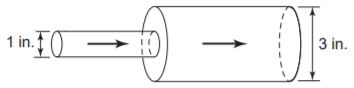• A. Its pressure decreases, but its speed increases.
• B. Its pressure and speed both decrease.
• C. Its pressure and speed both increase.
• D. Its pressure increases, but its speed decreases.

Total flow through the pipe must be the same everywhere, so water must flow faster in the 1-inch section. When a fluid moves faster, its pressure drops. Therefore, pressure is lower in the 1-inch pipe and rises as the water enters the 3-inch pipe.

6. What is the force F needed to balance this lever?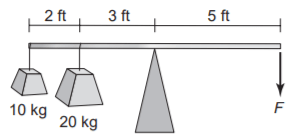• A. 20 kg
• B. 22 kg
• C. 25 kg
• D. 30 kg

To balance the lever, sum the moments of force
around the fulcrum:
(5 × 10 kg) + (3 × 20 kg) = 5F
50 + 60 = 5F
110 = 5F
F = 22

7. Gear A, with 48 teeth, meshes with gear B, with 12 teeth. For every rotation that gear A makes, how many rotations does gear B make?

• A. 1
• B. 2
• C. 4
• D. 8

Gear A, with 48 teeth, meshes with gear B, with 12 teeth. So for every complete rotation of gear A, gear B makes 48/12 = 4 complete rotations.

8. Pushing a heavy concrete block up an inclined plane will be easier if you

• A. raise the angle of the plane.
• B. turn the block upside down.
• C. heat the block with an electric coil.
• D. put the block on a wheelbarrow.

Of the choices, the only one that will make the job easier is to put the block on a wheelbarrow. The wheels will reduce friction and make it easier to move the block. Choice A, raising the angle of the plane, will decrease, not increase, the mechanical advantage. Choices B and C will have no effect.

9. These pipes and cylinders are part of a hydraulic mechanism. If the piston in cylinder A moves,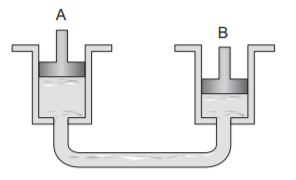• A. the piston in cylinder B will move.
• B. the fluid in cylinder A will not move.
• C. the piston in cylinder B will not move.
• D. the fluid in cylinder A will move, but the fluid in cylinder B will not move.

Choice A is correct because the hydraulic system transfers movement between the cylinders. The cylinders are directly linked and contain an incompressible fluid.

10. A 3 ft × 6 ft tank is filled with 3,600 lb of water. What is the pressure on the bottom, in lb/ft2?

• A. 100
• B. 200
• C. 400
• D. 800

The tank has an area of 18 square feet. Pressure  force/area. If the total weight of the water = 3,600 lb, weight per square foot =
3600/18 = 200 lb/ft2.

11. Which cannot bend without breaking?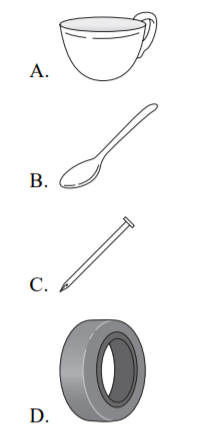Of the choices, only the teacup cannot bend without breaking. The spoon, nail, and automobile tire are all made of materials that can bend
without breaking.

12. A knife blade is an example of which kind of simple machine?

• A. Pulley
• B. Wheel and axle
• C. Inclined plane
• D. Gear

A knife blade is an example of an inclined plane.

13. In the pulley (sheave) system shown, pulley 1 is rotating at 80 rpm. How fast is pulley 2 rotating?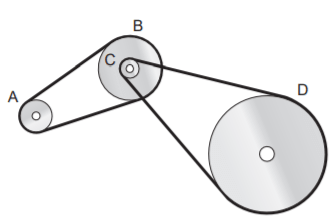• A. 180 rpm
• B. 240 rpm
• C. 300 rpm
• D. 360 rpm

The ratio of diameters determines the ratio of sheave speed. 6/2 = 3/1, so the driven sheave will rotate three times as fast as the drive sheave. 3 × 80 = 240.

14. In the pulley system shown (above), which pulley is rotating the slowest?

• A. A
• B. B
• C. C
• D. D

The pulley with the greatest diameter is pulley D. Therefore, it is the one that is rotating the slowest.

15. Operating this car jack will take less effort if you

• A. add weight to the other side of the car.
• B. get a longer jack handle.
• C. open the hood of the car.
• D. replace the jack handle with one just like it made of wood.

The car jack is a lever. Of the choices, the only one that will make the job easier is choice B, getting a longer jack handle. This will increase the effort distance in the lever and thereby increase the mechanical advantage.

16. Which material is best for the floor of a fireplace?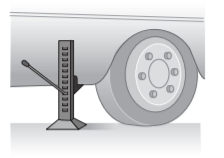• A. Wood
• B. Plastic
• C. Stone
• D. Glass

The floor of a fireplace must be made of a material that will not ignite or melt with the heat of the fire. Of the choices, the one that is best is stone.

17. What effort is required to lift the load?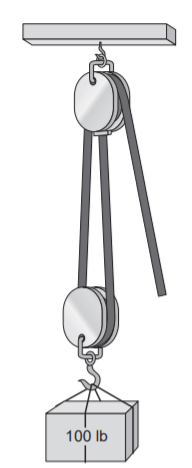• A. 40 lb
• B. 50 lb
• C. 100 lb
• D. 200 lb

Because the pulley has two supporting strands, it has a mechanical advantage of 2. Therefore, each pound of pull will produce 2 pounds of  lift.

18. What makes the water flow out of the tank and through the siphon hose into the bucket?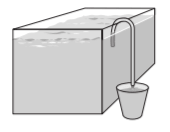• A. Air pressure
• B. Water pressure
• C. The weight of the water
• D. Magnetism

Air pressure is what makes a siphon function. The weight of the air on the surface of the water in the tank forces water through the hose and into the bucket.

19. In the diagram, gear B is an idler gear. If gear B is rotating clockwise,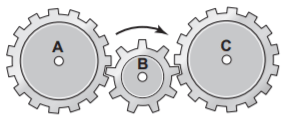• A. gear A is rotating clockwise, but gear C is rotating counterclockwise.
• B. gear A and gear C are rotating clockwise.
• C. gear A and gear C are rotating counterclockwise.
• D. gear A is rotating counterclockwise, but gear C is rotating clockwise.

To make two gears turn in the same direction, an idler gear is required between them. The idler gear turns in the opposite direction from the two gears on either side of it. If idler gear B is rotating clockwise, gears A and C are both rotating counterclockwise.

20. A wrench uses which simple machine to turn a bolt?

• A. Lever
• B. Inclined plane
• C. Pulley
• D. Wheel and axle

A wrench uses a wheel-and-axle effect to
move a bolt or nut.

21. The diagram shows a box of books on a shelf supported by two posts. Which post bears the greater part of the weight?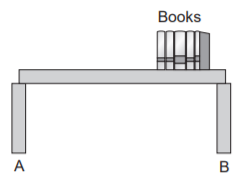• A. Post A.
• B. Post B.
• C. Each post bears the same amount of weight.
• D. The shelf distributes the weight equally.

The post nearer to the load bears the greater part of the weight. In the diagram, post B is the one nearer to the weight of the box of books.

22. Water is flowing into the tank from the upper faucet at the rate of 240 gallons per hour and draining out of the tank through the lower faucet at a rate of 1 gallon per minute. How many more gallons of water will be in the tank after 6 minutes?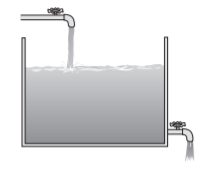• A. 14
• B. 16
• C. 18
• D. 22

Water is flowing into the tank at the rate of 240 gallons per hour, or 240/60 = 4 gallons per minute. Water is flowing out at the rate of 1 gallon per minute, so the net gain per minute is 3 gallons. In 6 minutes, the tank will gain 6 × 3 = 18 gallons of water.

23. The diagram shows a rotating disk. When the disk rotates, which point travels farthest?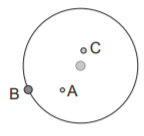• A. Point A.
• B. Point B.
• C. Point C.
• D. Points B and C both travel the same distance.

A point on the circumference must travel a longer distance than a point closer to the center.

24. Which flat cardboard pattern can be folded along the dotted lines to form the complete, totally enclosed box shown?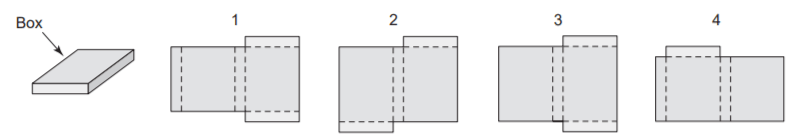• A. 1
• B. 2
• C. 3
• D. 4

Of the choices, the only one that will form the complete, totally enclosed box shown is pattern 1.

25. After the crank (A) rotates 9.5 times, where will the piston (B) be?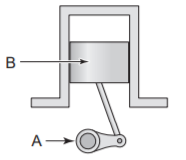• A. In the same place as shown
• B. At the top of the cylinder
• C. At the bottom of the cylinder
• D. B or C
 Document Type Download Link Free Editable Doc File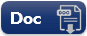Free Printable PDF File